## Chirality

CHIRALITY IN OPTICAL FIELDS

KEY to Table Symbols:

• 1-DE with left orientation (l) top This is sometimes referred to as 'Left Hingeing'
• 1-DE with right orientation (r)bottom This is sometimes referred to as 'Right Hingeing'• External arrows: denote spin chirality of field. A=anticlockwise, C=clockwise, N=neutral.
• Internal arrows: denote inclination of elements to field centre. f=centrifugal, p=centripetal, n=neutral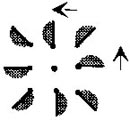[An]r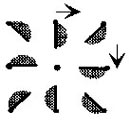[Cn]r[Nf]r[Np]r[An]l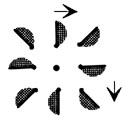[Cn]l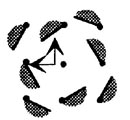[Nf]l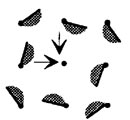[Np]l[Cp]l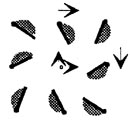[Cf]l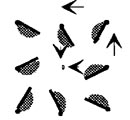[Ap]r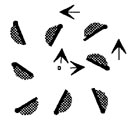[Af]r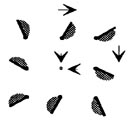[Cp]r[Cf]r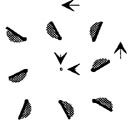[Ap]l[Af]l
If a field is either an [N] or an [n] type the effect is the same for fields composed of elements that are either 'left oriented' or 'right oriented
so that using (l) and (r) hingeing there are a total of 16 types of field. When the actual field effects are analysed it is found that 8 types exist.
as the chirality of the 1-D elements can be discounted except at very close range. Then it becomes merely a matter of field diameter for the
number of elements in the respective field

.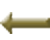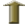Last Revised: 30/12/99